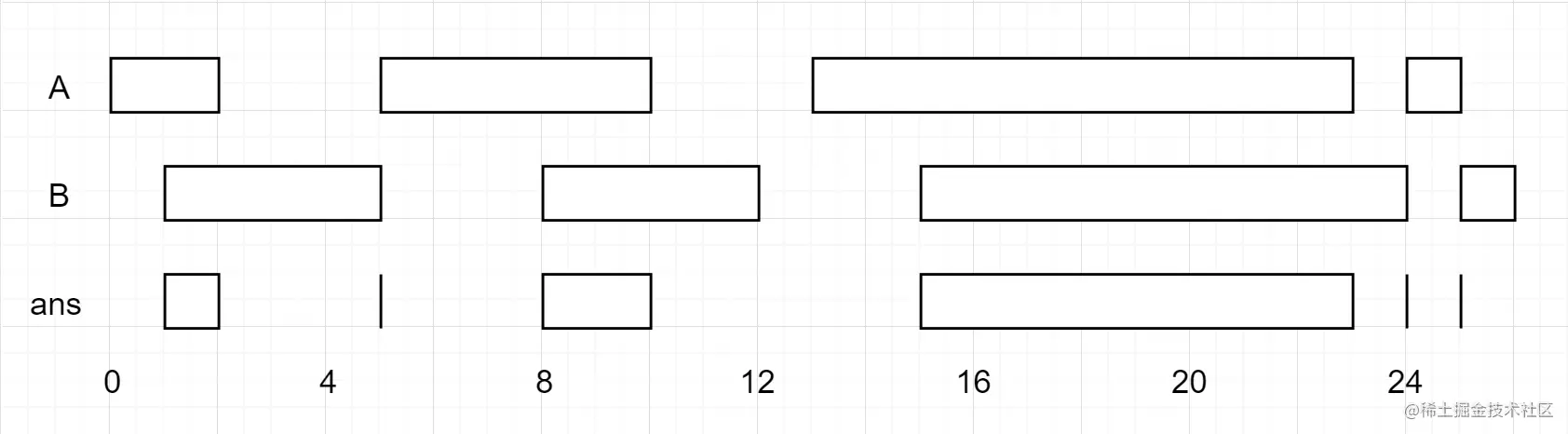# leetcode 986. Interval List Intersections（python）

2022-01-31 19:01:55

「 This is my participation 11 The fourth of the yuegengwen challenge 18 God , Check out the activity details ：2021 One last more challenge

### describe

You are given two lists of closed intervals, firstList and secondList, where firstList[i] = [starti, endi] and secondList[j] = [startj, endj]. Each list of intervals is pairwise disjoint and in sorted order.

Return the intersection of these two interval lists.

A closed interval [a, b] (with a <= b) denotes the set of real numbers x with a <= x <= b.

The intersection of two closed intervals is a set of real numbers that are either empty or represented as a closed interval. For example, the intersection of [1, 3] and [2, 4] is [2, 3].

Example 1:``````Input: firstList = [[0,2],[5,10],[13,23],[24,25]], secondList = [[1,5],[8,12],[15,24],[25,26]]
Output: [[1,2],[5,5],[8,10],[15,23],[24,24],[25,25]]
Copy code ``````

Example 2:

``````Input: firstList = [[1,3],[5,9]], secondList = []
Output: []
Copy code ``````

Example 3:

``````Input: firstList = [], secondList = [[4,8],[10,12]]
Output: []
Copy code ``````

Example 4:

``````Input: firstList = [[1,7]], secondList = [[3,10]]
Output: [[3,7]]
Copy code ``````

Note:

• 0 <= firstList.length, secondList.length <= 1000
• firstList.length + secondList.length >= 1
• 0 <= starti < endi <= 109
• endi < starti+1
• 0 <= startj < endj <= 109
• endj < startj+1

### analysis

According to the meaning , Given two closed interval lists ,firstList and secondList, among firstList[i] = [starti, endi] and secondList[j] = [startj, endj]. Each interval list is disjoint in pairs , And sort them in order . The title asks us to return the intersection of these two interval lists .

The concepts of closed interval and intersection are also given in the title , Closed interval [a, b]（a <= b） For real numbers x Set ,a <= x <= b. The intersection of two closed intervals is a set of real numbers , They are either empty , Or expressed as a closed interval . for example ,[1, 3] and [2, 4] The intersection of [2, 3].

The idea is simple , Directly traverse all closed intervals to find the intersection ：

• If A perhaps B There is an empty list , Return the empty list directly

• Initialize two pointers i and j All for 0 , result result Empty list

• When i Less than A And j Less than B The length of time has been carried out while loop

``````   If  A[i] < B[j]  explain  A[i]  and  B[j]  There is no intersection ,i  Add one for the next cycle
Empathy  B[j] < A[i]  Also explain   A[i]  and  B[j]  There is no intersection  ,j  Add one for the next cycle
If there is an intersection , Deposit it directly into  result  in
If  A[i] < B[j]  Description needs to be viewed  A[i+1]  And  B[j]  Whether there is an intersection , therefore   i  Add one , otherwise  j  Add one , The same thing
Copy code ``````
• Return to the end of the loop result that will do .

``````class Solution(object):
def intervalIntersection(self, A, B):
"""
:type A: List[List[int]]
:type B: List[List[int]]
:rtype: List[List[int]]
"""
if not A or not B: return []
i = j = 0
result = []
while i < len(A) and j < len(B):
if A[i] < B[j]:
i += 1
continue
if B[j] < A[i]:
j += 1
continue
result.append([max(A[i], B[j]), min(A[i], B[j])])
if A[i] < B[j]:
i += 1
else:
j += 1
return result

Copy code ``````

### Running results

``````Runtime: 116 ms, faster than 90.07% of Python online submissions for Interval List Intersections.
Memory Usage: 14.3 MB, less than 69.98% of Python online submissions for Interval List Intersections.
Copy code ``````

### analysis

You can also simplify the code , The principle of same .

``````class Solution(object):
def intervalIntersection(self, A, B):
"""
:type A: List[List[int]]
:type B: List[List[int]]
:rtype: List[List[int]]
"""
if not A or not B: return []
i = j = 0
result = []
while i < len(A) and j < len(B):
L = max(A[i], B[j])
R = min(A[i], B[j])
if L<=R:
result.append([L, R])
if A[i] < B[j]:
i += 1
else:
j += 1
return result
Copy code ``````

### Running results

``````Runtime: 112 ms, faster than 96.69% of Python online submissions for Interval List Intersections.
Memory Usage: 14.3 MB, less than 69.98% of Python online submissions for Interval List Intersections.
Copy code ``````